Latest Banking jobs   »

# Quantitative Aptitude Quiz For IRDA AM 2023 – 10th May

Q1. A, B and C start simultaneously from X to Y. A reaches Y, turns back and meet B at a distance of 11 km from Y. B reached Y, turns back and meet C at a distance of 9 km from Y. If the ratio of the speeds of A and C is 3:2, what is the distance between X and Y (in km)?
(a) 108
(b) 198
(c) 90
(d) 99
(e) None of these

Q2. Two cars A and B travel a distance of x km and x+200 km respectively. Ratio of speed of A and B is 3 : 4 and total time taken by A and B in covering distance of x and x+200 km respectively is in the ratio 2 : 3 then find the sum of total distance covered by both cars.
(a) 1200 km
(b) 300 km
(c) 800 km
(d) 600 km
(e) 500 km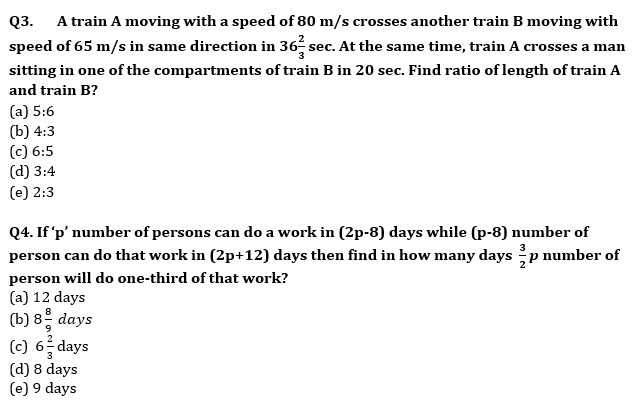Q5. 8 men and 4 women together can complete a piece of work in 6 days. Work done by a man in one day is double the work done by a woman in one day. If 8 men and 4 women started working and after 2 days, 4 men left and 4 new women joined, in how many more days will the work be completed?
(a) 5 days
(b) 8 days
(c) 6 days
(d) 4 days
(e) None of these

Q6. A train ‘P’ crosses a pole in 6.75 sec and a 240-meter-long platform in 15.75 sec. If train ‘Q’ which is 120-meter-long running in same direction crosses train ‘P’ in 45 sec, then find time required by train ‘Q’ to cross train ‘P’ running in opposite direction?
(a) 6sec
(b) 7sec
(c) 5 sec
(d) 9 sec
(e) 10 sec

Q7. Speed of a boat in still water is 8 km/h. It takes 5 hours to go upstream and 3 hours downstream distance between two points. What is the speed of stream?
(a) 4 km/h
(b) 2 km/h
(c) 3 km/h
(d) 1 km/h
(e) 2.5 km/h

Q8. Two boats A and B are 120 km apart in a river and they start to move towards each other and meet after 6 hours. If ratio of speed of boat in still water to downstream speed of boat is 5 : 6, then find the speed of boat in still water. (consider speed of both boat in still water is same)
(a) 9 km/hr
(b) 7 km/hr
(c) 6 km/hr
(d) 8 km/hr
(e) 10 km/hr
Q9. Pipe P₁ can fill ⅗ th of the tank in 9 minutes. There are two more pipes P₂ and P₃, in which P₂ is 50% more efficient than P₁ and P₃ pipe is 5/9 th as efficient as P₂. Then calculate the time taken by all the three pipes to fill that tank when opened together?
(a) 5 min
(b) 6 min
(c) 7 ½ min
(d) 3 ½ min
(e) 4 ½ min

Q10. A cistern has two pipes. One can fill it with water in 15 hours and other can empty it in 12 hours. In how many hours will the cistern be emptied if both the pipes are opened together when 3/5 th of the cistern is already full of water?
(a)36 hr
(b)24 hr
(c)60 hr
(d)45 hr
(e)30 hr

Q11. On Rs 3500 invested at a simple interest rate 7 per cent per annum, Rs 500 is obtained as interest in certain years, In order to earn Rs 800 as interest on Rs 4900 in the same number of years, what should be the rate of simple interest?
(a) 9%
(b) 10%
(c) 12%
(d) 8%
(e) None of these

Q12. A shopkeeper gives a discount of 20% on marked price of article A and C.P. of another article B is 20% more than selling price of article A. If shopkeeper sold article B at 15% profit & S.P. of article B is Rs 1216 more than SP of article A. Then find M.P of article A?
(a) Rs 3000
(b) Rs 3500
(c) Rs 4500
(d) Rs 3200
(e) Rs 4000

Q13. Ratio between cost price and marked price of an article is 5 :7 and shopkeeper allows two successive discounts of 15% and 12% on marked price. If shopkeeper made a profit of Rs.354, then find at what price shopkeeper should sold the article to make a profit of 20%?
(a) 8400 Rs.
(b) 9600 Rs.
(c) 10200 Rs.
(d) 9000 Rs.
(e) 11600 Rs.

Q14. Average marks of a student in five subjects is 80. If the average marks of first two subject is 84.5 and the average marks of last two subject is 83. Find the marks of third subject?
(a) 51
(b) 53
(c) 65
(d) 63
(e) 60

Q15.The average age of 8 students in a college is 22.5 years. When two new students joined them, the average is increased by 2.5 years. Again, when another two new students are included the average is further increased by 1 year. Find the average age of all new students.
(a) 33 yr
(b) 32 yr
(c) 30 yr
(d) 34 yr
(e) 35 yr

Solutions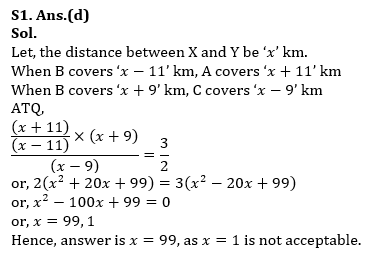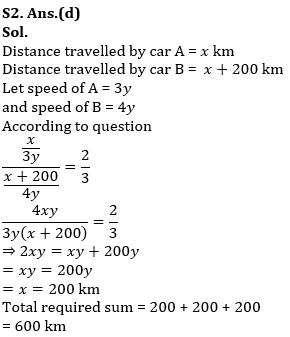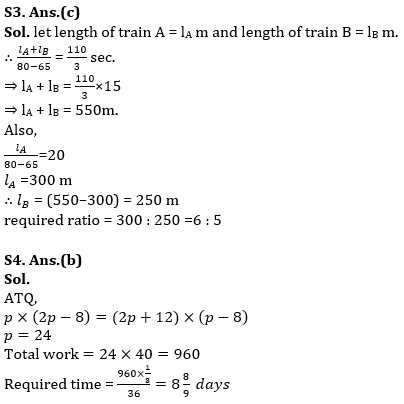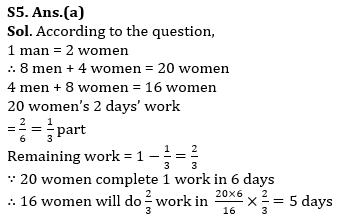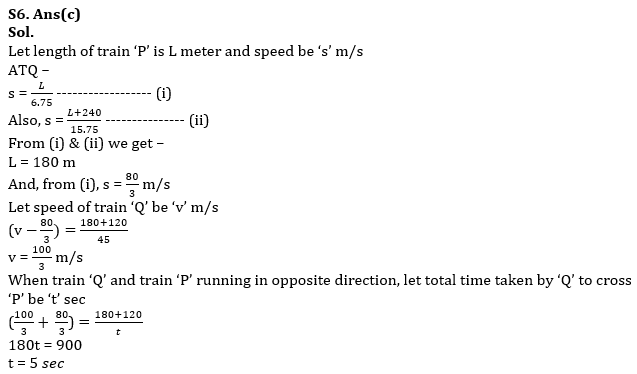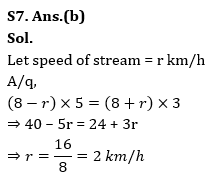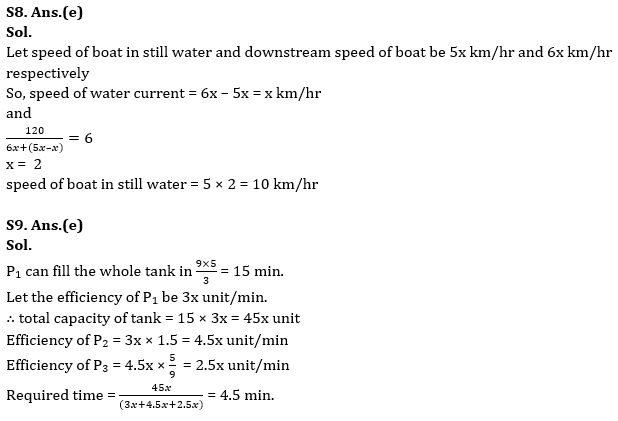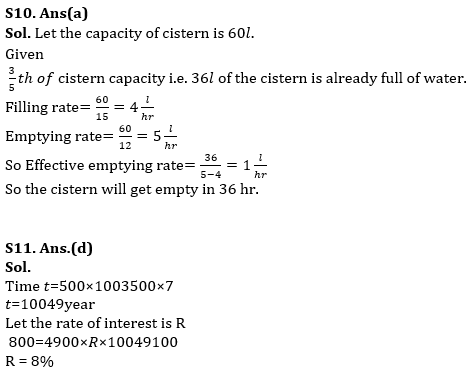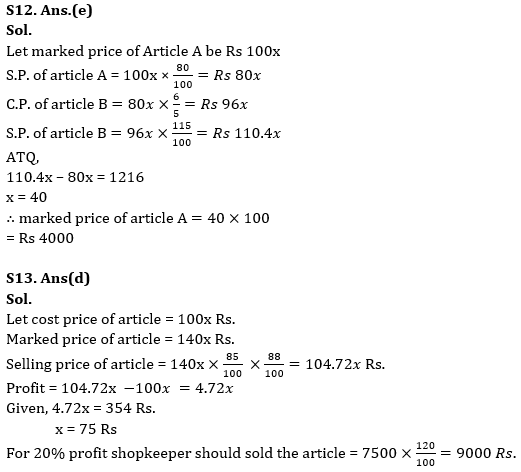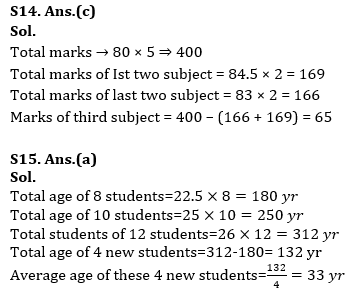## FAQs

### How many sections are there in the IRDAI exam?

There are 4 sections in the IRDAI assistant manager online preliminary exam. They are Reasoning, English Language, General awareness, Quantitative aptitude.

#### Congratulations!Union Budget 2023-24: Free PDF